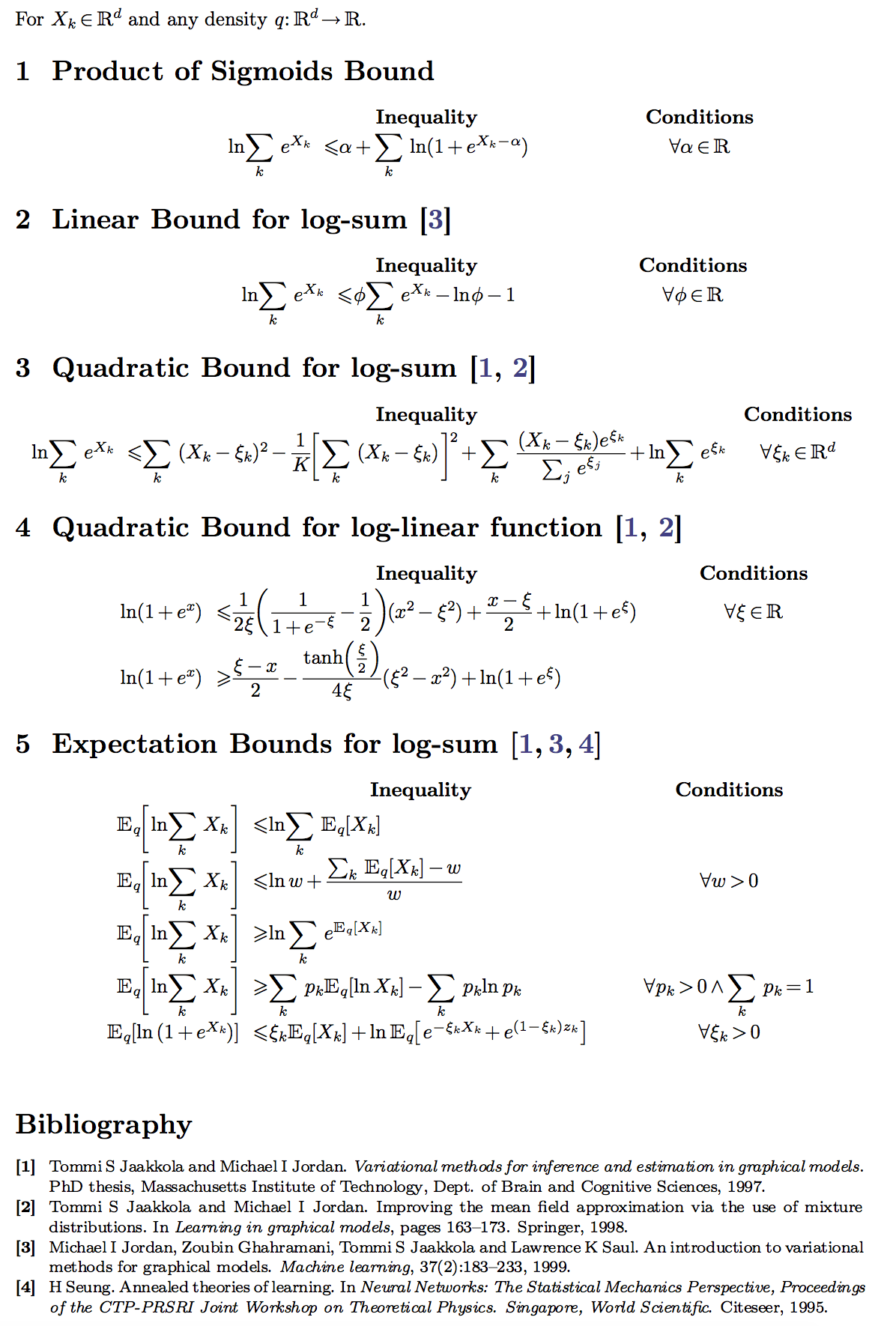# Useful Inequalities for Variational Inference

Variational Inference is a technique which consists in bounding the log-likelihood ln p(x) defined by a model with latent variables p(x,z)=p(x|z)p(z) through the introduction of  a variational distribution q(z|x) with same support as p(z):Often the expectations in the bound F(x) (aka, ELBO or Free Energy) cannot be solved analytically.

In some cases, we can make use of a few handful inequalities which I quickly summarize below.

Some of these inequalities introduce new variational parameters. Those should be optimized jointly with all the other parameters to minimize the ELBO.## 3 thoughts on “Useful Inequalities for Variational Inference”

This site uses Akismet to reduce spam. Learn how your comment data is processed.